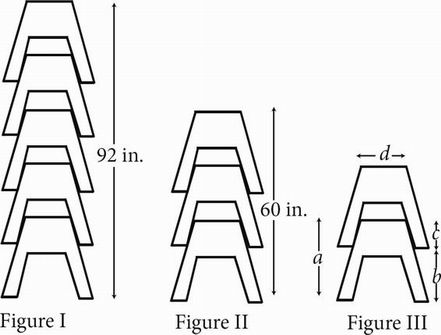# SAT Math Multiple Choice Question 232: Answer and Explanation

### Test Information

Question: 232

7.In figures I and II above, two stacks of identical carpenter's sawhorses are shown, with heights of 92 and 60 inches, respectively. The height, in inches, of a stack of k sawhorses is given by the function h(k) = 16k + 12, where k is a positive integer and k ≥ 1. The number 12 in the function represents which of the dimensions shown in Figure III ?

• A. a, the height of one sawhorse
• B. b, the distance from the bottom of one sawhorse to the bottom of the next highest sawhorse
• C. c, the distance from the top of one sawhorse to the bottom of the next highest sawhorse
• D. d, the width of a sawhorse at the top